This example shows how to use an RLS filter to extract useful information from a noisy signal. The information bearing signal is a sine wave that is corrupted by additive white gaussian noise.

The adaptive noise cancellation system assumes the use of two microphones. A primary microphone picks up the noisy input signal, while a secondary microphone receives noise that is uncorrelated to the information bearing signal, but is correlated to the noise picked up by the primary microphone.

Note: This example is equivalent to the Simulink® model 'rlsdemo' provided.

The model illustrates the ability of the Adaptive RLS filter to extract useful information from a noisy signal.

priv_drawrlsdemo axis off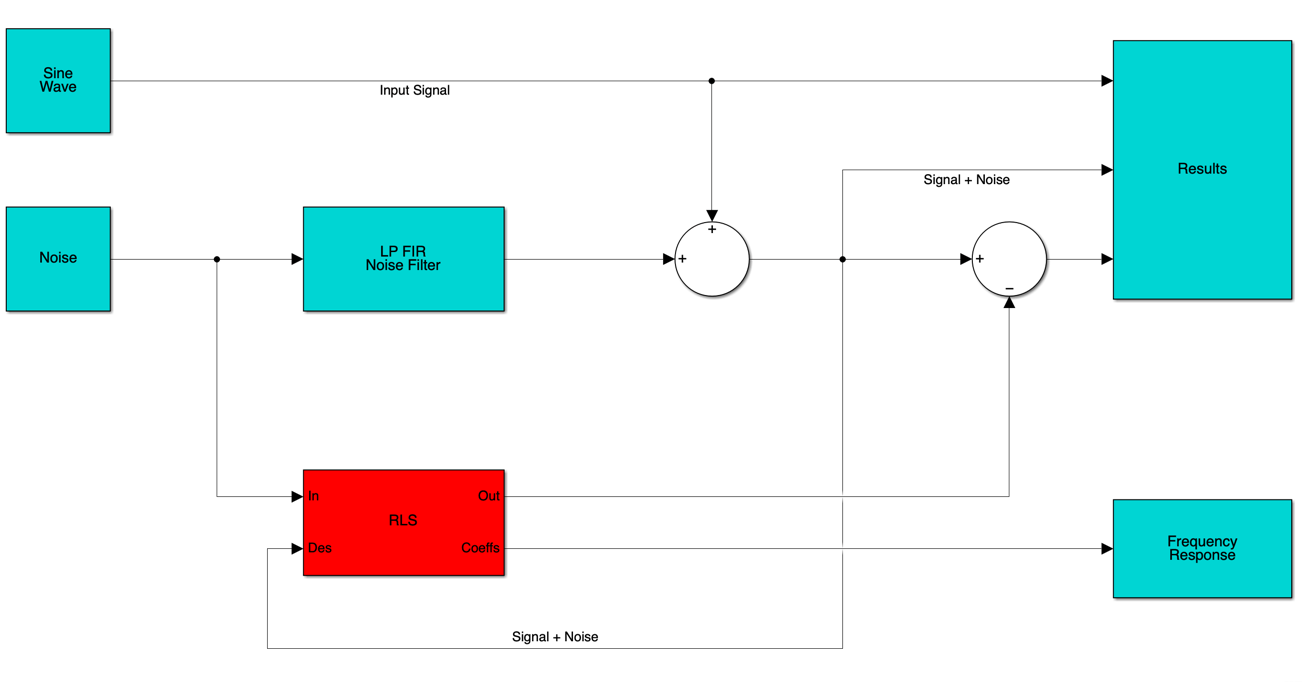The information bearing signal is a sine wave of 0.055 cycles/sample.

signal = sin(2*pi*0.055*(0:1000-1)'); signalSource = dsp.SignalSource(signal,'SamplesPerFrame',100,... 'SignalEndAction','Cyclic repetition'); plot(0:199,signal(1:200)); grid; axis([0 200 -2 2]); title('The information bearing signal');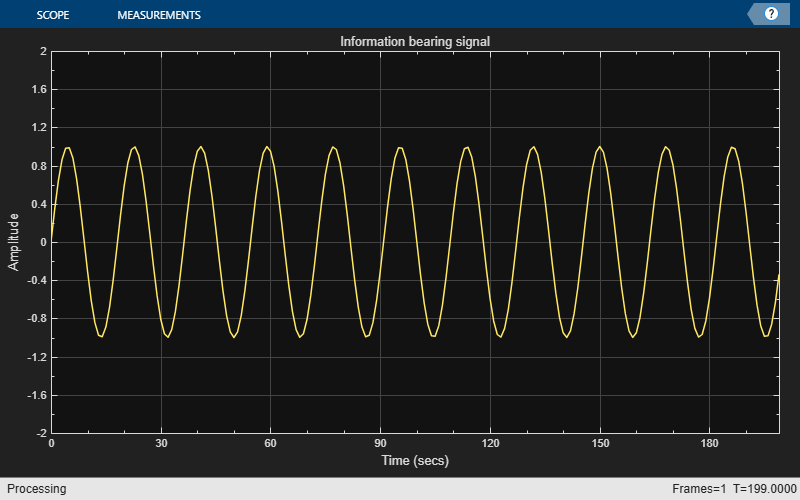The noise picked up by the secondary microphone is the input for the RLS adaptive filter. The noise that corrupts the sine wave is a lowpass filtered version of (correlated to) this noise. The sum of the filtered noise and the information bearing signal is the desired signal for the adaptive filter.

nvar = 1.0; % Noise variance noise = randn(1000,1)*nvar; % White noise noiseSource = dsp.SignalSource(noise,'SamplesPerFrame',100,... 'SignalEndAction','Cyclic repetition'); plot(0:999,noise); title('Noise picked up by the secondary microphone'); grid; axis([0 1000 -4 4]);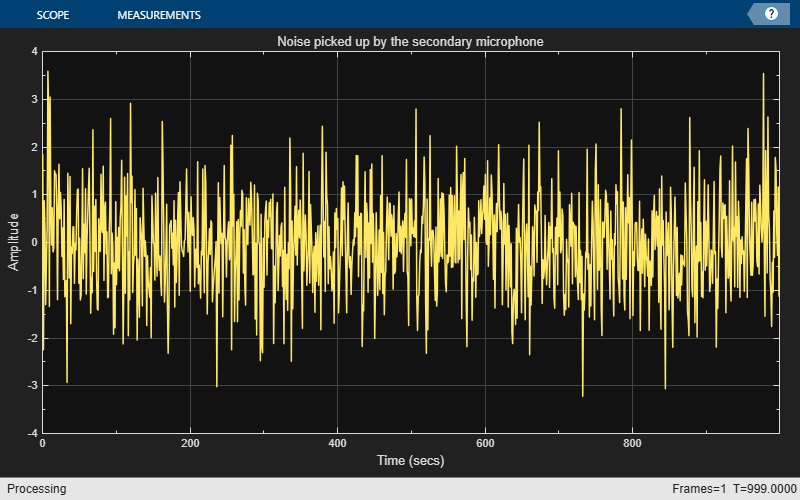The noise corrupting the information bearing signal is a filtered version of 'noise'. Initialize the filter that operates on the noise.

lp = dsp.FIRFilter('Numerator',fir1(31,0.5));% Low pass FIR filter

Set and initialize RLS adaptive filter parameters and values:

M = 32; % Filter order delta = 0.1; % Initial input covariance estimate P0 = (1/delta)*eye(M,M); % Initial setting for the P matrix rlsfilt = dsp.RLSFilter(M,'InitialInverseCovariance',P0);

Running the RLS adaptive filter for 1000 iterations. As the adaptive filter converges, the filtered noise should be completely subtracted from the "signal + noise". Also the error, 'e', should contain only the original signal.

scope = timescope('TimeSpan',1000,'YLimits',[-2,2], ... 'TimeSpanOverrunAction','Scroll'); for k = 1:10 n = noiseSource(); % Noise s = signalSource(); d = lp(n) + s; [y,e] = rlsfilt(n,d); scope([s,e]); end release(scope);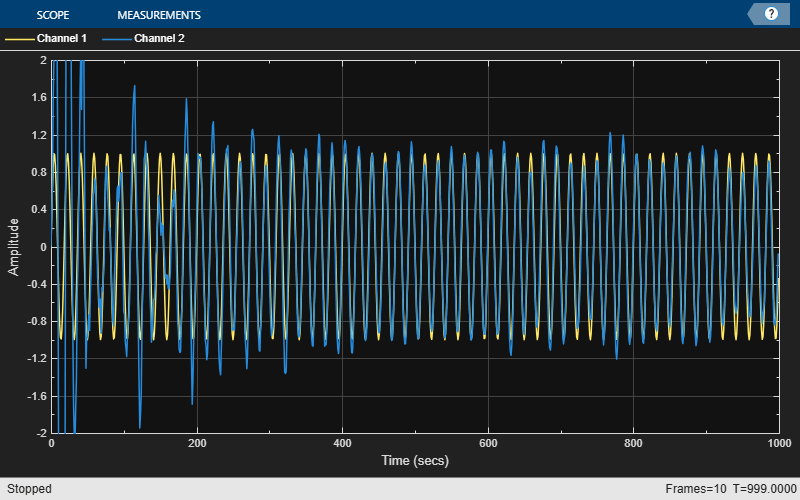The plot shows the convergence of the adaptive filter response to the response of the FIR filter.

H = abs(freqz(rlsfilt.Coefficients,1,64)); H1 = abs(freqz(lp.Numerator,1,64)); wf = linspace(0,1,64); plot(wf,H,wf,H1); xlabel('Normalized Frequency (\times\pi rad/sample)'); ylabel('Magnitude'); legend('Adaptive Filter Response','Required Filter Response'); grid; axis([0 1 0 2]);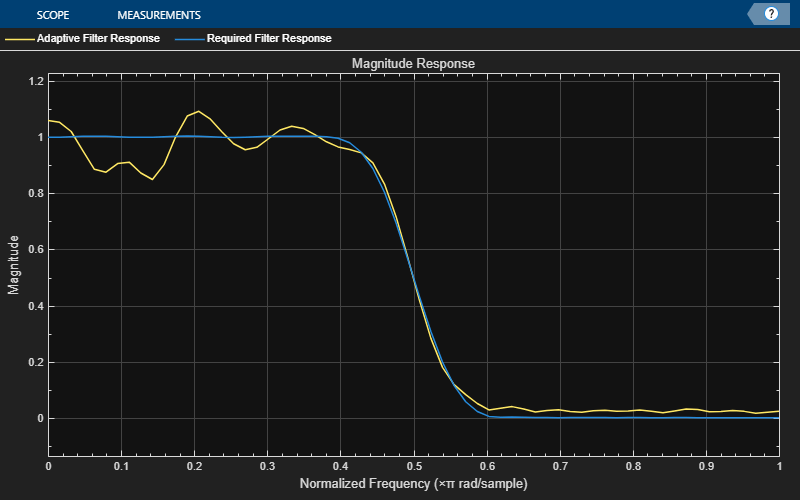S.Haykin, "Adaptive Filter Theory", 3rd Edition, Prentice Hall, N.J., 1996.